Function Repository Resource:

# SequenceGraph

Create a graph from a data sequence

Contributed by: Daniel de Souza Carvalho
 ResourceFunction["SequenceGraph"][list] creates a graph connecting adjacent entries of list.

## Details and Options

In ResourceFunction["SequenceGraph"][list], list can contain numbers, strings or symbolic values.
ResourceFunction["SequenceGraph"] accepts the same options as Graph.

## Examples

### Basic Examples (1)

Plot a simple series of numbers:

 In:=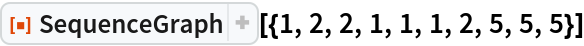Out=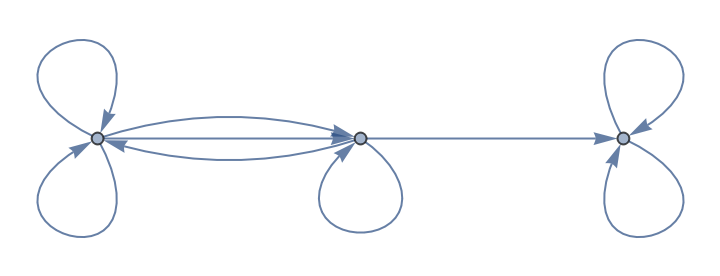### Scope (5)

SequenceGraph accepts string elements as well as any options accepted by Graph:

 In:=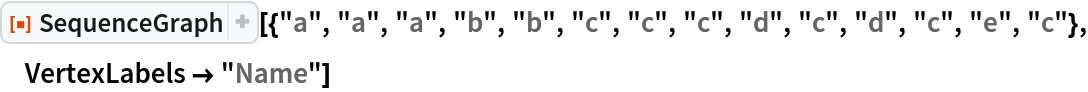Out=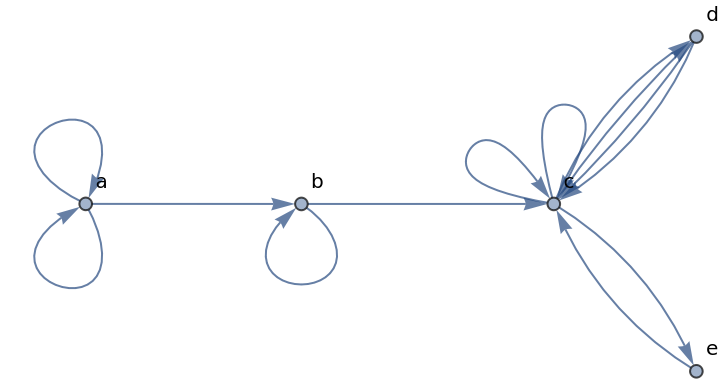SequenceGraph accepts a series of symbolic values:

 In:=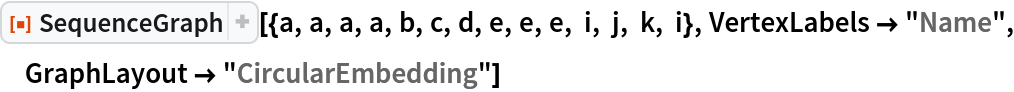Out=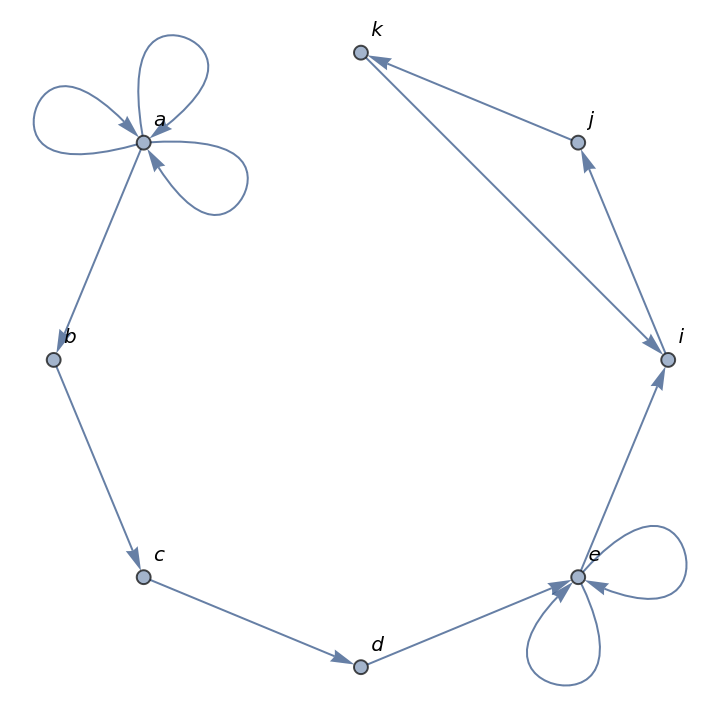Plot a series created by a range of data:

 In:=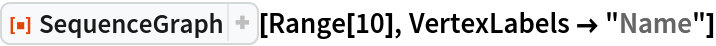Out=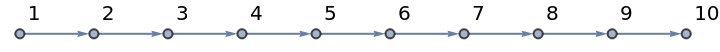Plot the digits of π:

 In:=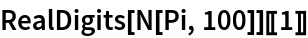Out=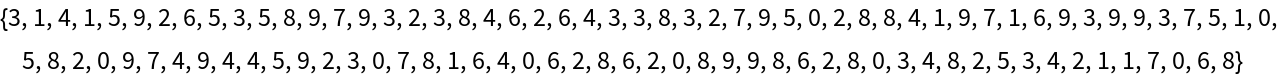In:=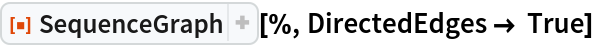Out=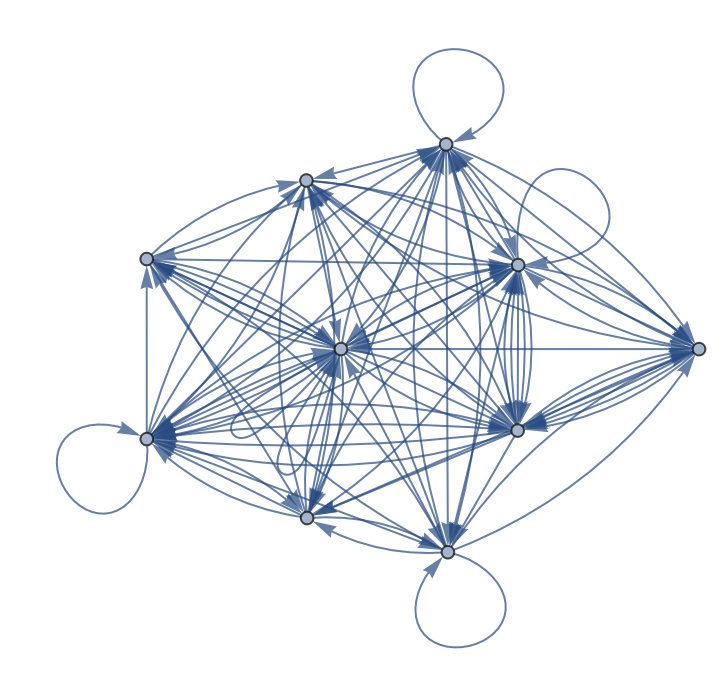Plot the digits of :

 In:=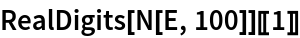Out=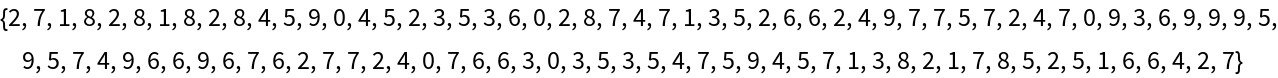In:=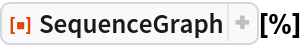Out=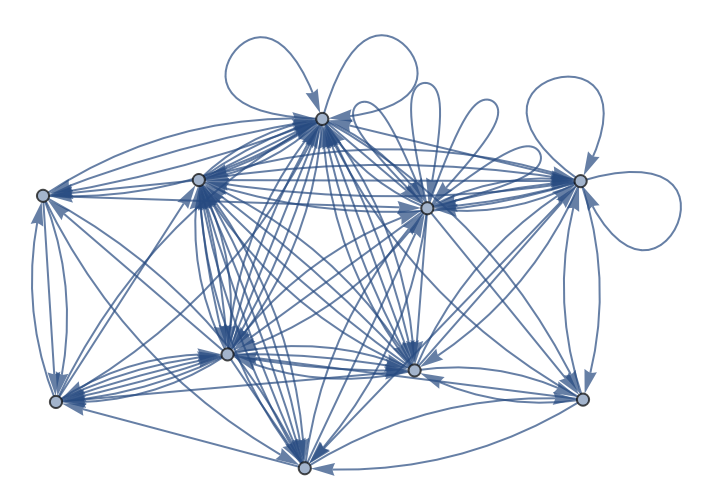### Applications (3)

Analyze the dynamical behavior of a class-4 cellular automaton by the sum of black cells of each step, and view as a graph:

 In:=Out=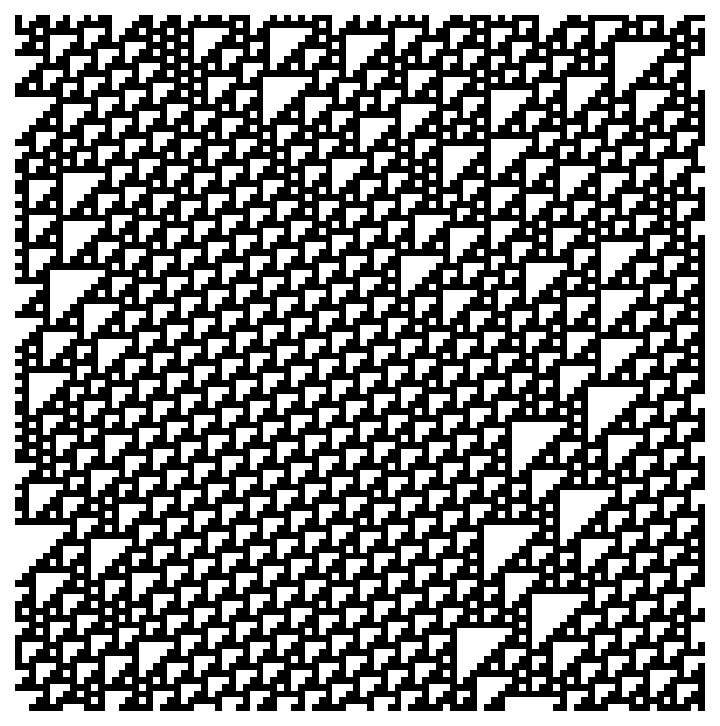In:=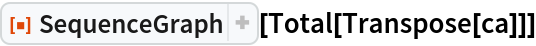Out=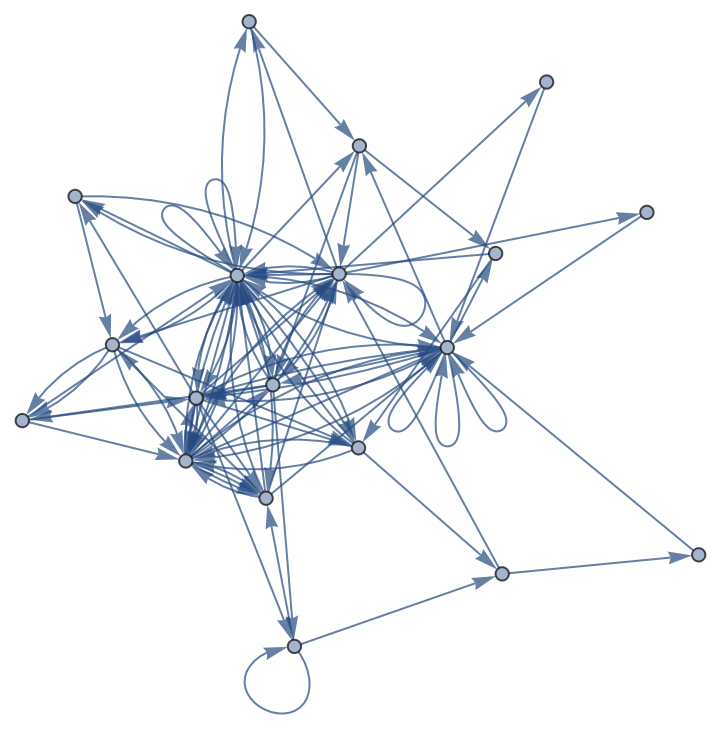Analyze the dynamical behavior of a class-2 cellular automaton by the sum of black cells of each step, and view as a graph:

 In:=Out=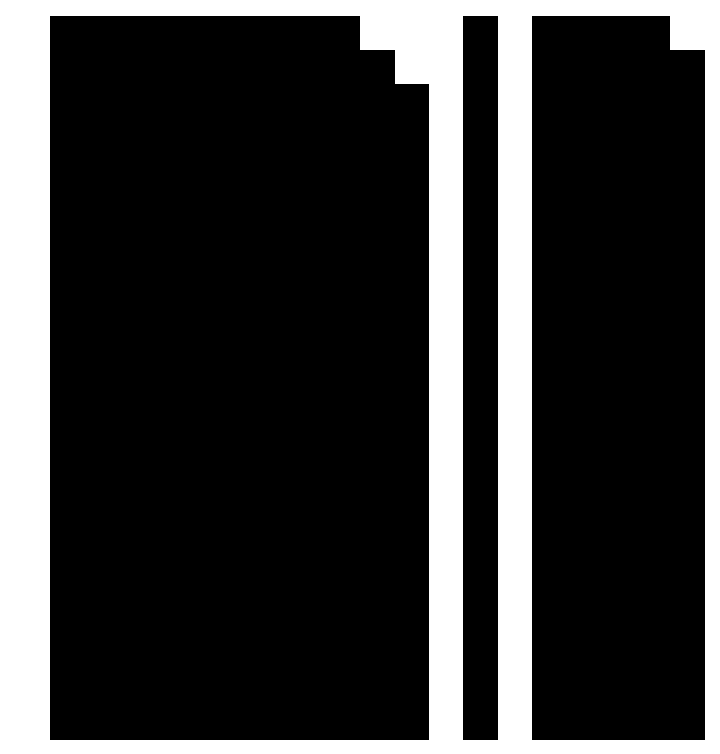In:=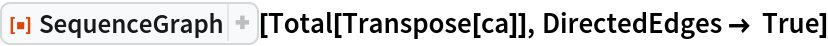Out=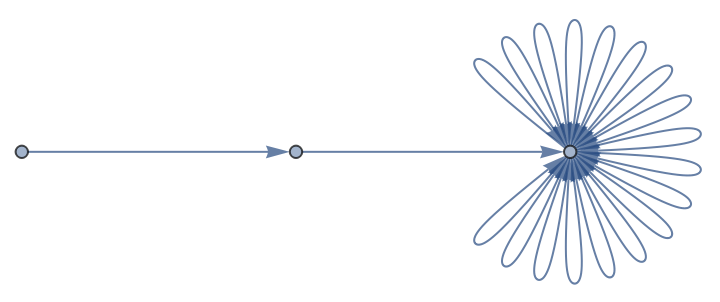Plot the sequence of words in a book:

 In:=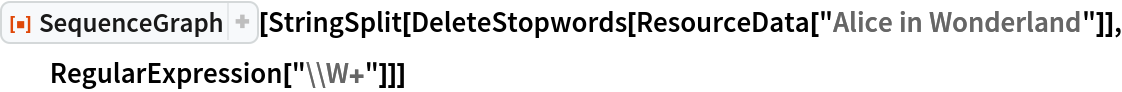Out=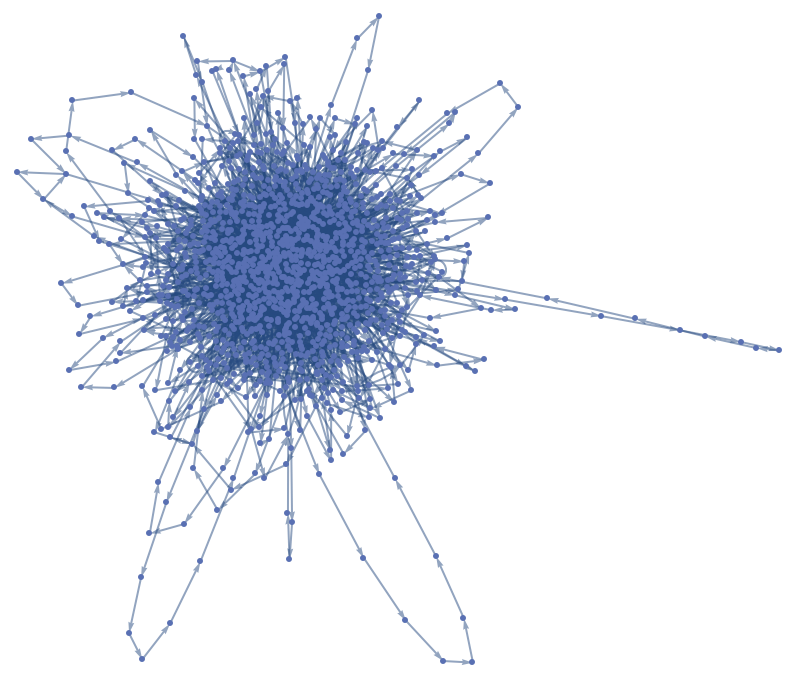## Publisher

Daniel de Souza Carvalho

## Version History

• 1.0.0 – 24 September 2019